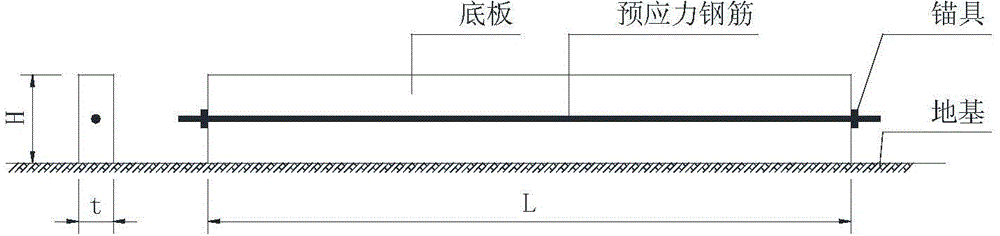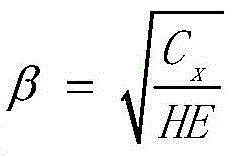# Computing method of secondary axis force of prestress bottom plate

## A technology of prestressed base plate and calculation method, which is applied in the direction of calculation, special data processing application, instrument, etc., can solve the problem that the secondary axial force cannot be calculated, and the influence of the secondary axial force cannot be accurately predicted.

Inactive Publication Date: 2015-01-28

3 Cites 4 Cited by

## AI-Extracted Technical Summary

### Problems solved by technology

 The purpose of the present invention is to overcome the problem that the secondary axial force cannot be calculated in complex prestressed concrete structures such as the prestressed floor in the prior art, so that the influence of the secondary axial force on co...
View more

## Abstract

The invention discloses a computing method of a secondary axis force of a prestress bottom plate. The computing method includes 1) computing equivalent precompression Npc0 of end concrete of the bottom plate according to tension control stress and area of end prestressed reinforcing bars of the prestress bottom plate; 2) computing coefficient beta according to the thickness H and the length L of the bottom plate, the foundation horizontal resistant coefficient Cx and the concrete elastic modulus E; 3) computing the secondary axis force Nr of the prestress bottom plate, wherein the unit of the Nr is N. The computing method is used for computing the secondary axis force caused by the foundation horizontal resistance, and with the computing formula given in this method, the secondary axis force of various sections in the concrete prestress bottom plate can be conveniently computed, and sufficient reference can be provided for engineering design and computing.

Application Domain

Special data processing applications

Technology Topic

RebarElastic modulus +5

## Image

•••## Examples

• Experimental program(1)

### Example Embodiment

 The specific embodiments of the present invention will be described below in conjunction with the drawings. The calculation formula of the secondary axial force caused by the horizontal resistance of the foundation is derived as follows:
 The thickness of the prestressed bottom plate is much smaller than the dimensions (length and width) in the other two directions, and the force calculation model of a long slab on the elastic foundation is established (see figure 1 ), the action of the prestressed steel bar is equivalent to the pressure N pc0.
 Due to the existence of the horizontal resistance of the foundation, the shear stress of the foundation acts on the bottom plate, and its magnitude is:
 τ=-C x u
 Where τ—shear stress on the contact surface between the bottom plate and the foundation (MPa);
 u—The horizontal displacement of the foundation at the shear stress, that is, the horizontal displacement of the bottom plate (mm);
 C x -Ground level resistance coefficient (N/mm 3 ), the negative sign means that the direction of the shear stress is always opposite to the displacement.
 Such as figure 2 As shown, at any point x of the bottom plate, a section of microbody with a length of dx is cut, the height of the microbody is taken as the full height H, its thickness is the unit thickness, and the displacement is u. The compressive stress on the left and right sides of the microbody is the effective prestress of the prestressed steel bar for the longevity of the bottom plate, respectively N x , N x +dN x. The bottom is the shear stress τ of the foundation to the bottom plate. Take the horizontal projection and list the balance equation ∑x=0:
 N x +dN x -N x +τdx=0, that is:
 dN x +τdx=0
 by: (E is the elastic modulus of concrete), τ=-C x u, get:
 E d 2 u dx 2 - C x u H = 0
 Assume C x HE = β , then:
 d 2 u dx 2 - β 2 u = 0
 The general solution of this differential equation:
 μ=Ach(βx)+Bsh(βx)
 From the boundary conditions x=0, u=0, Ach(0)=0, A=0
 By boundary conditions x = L 2 , N x = N pc 0 , dμ dx = Bβch ( βx ) Get:
 EBβch ( β L 2 ) H = N pc 0
 B = N pc 0 HEβch ( β L 2 )
 Therefore,
 u = N pc 0 HEβch ( β L 2 ) sh ( βx )
 N x = ch ( βx ) ch ( β L 2 ) N pc 0
 The secondary axial force of the prestressed floor caused by the horizontal resistance of the foundation N r :
 N r = N x - N pc 0 = ( ch ( βx ) ch ( β L 2 ) - 1 ) N pc 0
 In the embodiment, the pre-stressed bottom plate has a thickness of 1000 mm and a length of 30,000 mm, and is located on the soil foundation. According to the geological survey report, the ground level resistance coefficient C x =5×10 -2 N/mm3, the bottom plate adopts C30 concrete, the elastic modulus E=3×10 4 N/mm2. In order to control the crack width of the bottom plate, prestress is applied to the middle of the bottom plate, and the end unit thickness is equivalent to N pc0 =2000N, find the secondary axial force of each section of the bottom plate caused by the horizontal resistance of the foundation.
 β = C x HE = 5 X 10 - 2 1000 X 3 X 10 4 = 4.08 X 10 - 5
 See Table 1 below for the secondary axial force of each control section.
 Table 1

 Of course, the above description does not limit the present invention, and the present invention is not limited to the above examples. Changes, modifications, additions or substitutions made by those skilled in the art within the essential scope of the present invention also belong to the present invention. protected range.

## PUM## Description & Claims & Application Information

We can also present the details of the Description, Claims and Application information to help users get a comprehensive understanding of the technical details of the patent, such as background art, summary of invention, brief description of drawings, description of embodiments, and other original content. On the other hand, users can also determine the specific scope of protection of the technology through the list of claims; as well as understand the changes in the life cycle of the technology with the presentation of the patent timeline. Login to view more.
Who we serve
• R&D Engineer
• R&D Manager
• IP Professional
Why Eureka